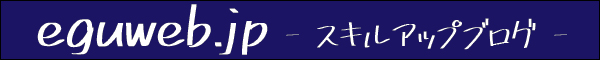# [FILTER function] How to perform a “fuzzy search” in combination with the FIND function (Google Spreadsheet)

This is a method for fuzzy search (extraction) with the FILTER function.

## What is the FILTER function?

You can use the FILTER function to extract the result of a specific condition from within a range.

``=FILTER(array, contains, [if empty])``

By using the FILTER function of Google Sheets, you can extract the result of the specified value from the table.

I was able to extract the ring chrysanthemum (white) in row F.

## Is fuzzy search not possible with the FILTER function?

Even if you try to do a fuzzy search with this filter function, the result will not be returned as it is. Is it possible with asterisk? And even if I try, it will return an error (no matches).

``=FILTER(estimate_quantity_flower!A:K,estimate_quantity_flower!F:F="輪菊*")``

In this case, it is a method to realize a fuzzy search.

## Combining FIND functions

Now combine the FIND function.

``=FILTER(estimate_quantity_flower!A:K,FIND("輪菊",estimate_quantity_flower!F:F))``

The fuzzy search returned results!

## summary

Combining the FIND function with the FILTER function changed it to “contains”.

Why is the return value of the FIND function like this…? is a mystery at the moment, but by combining the FILTER function and the FIND function, it is possible to extract fuzzy filters.